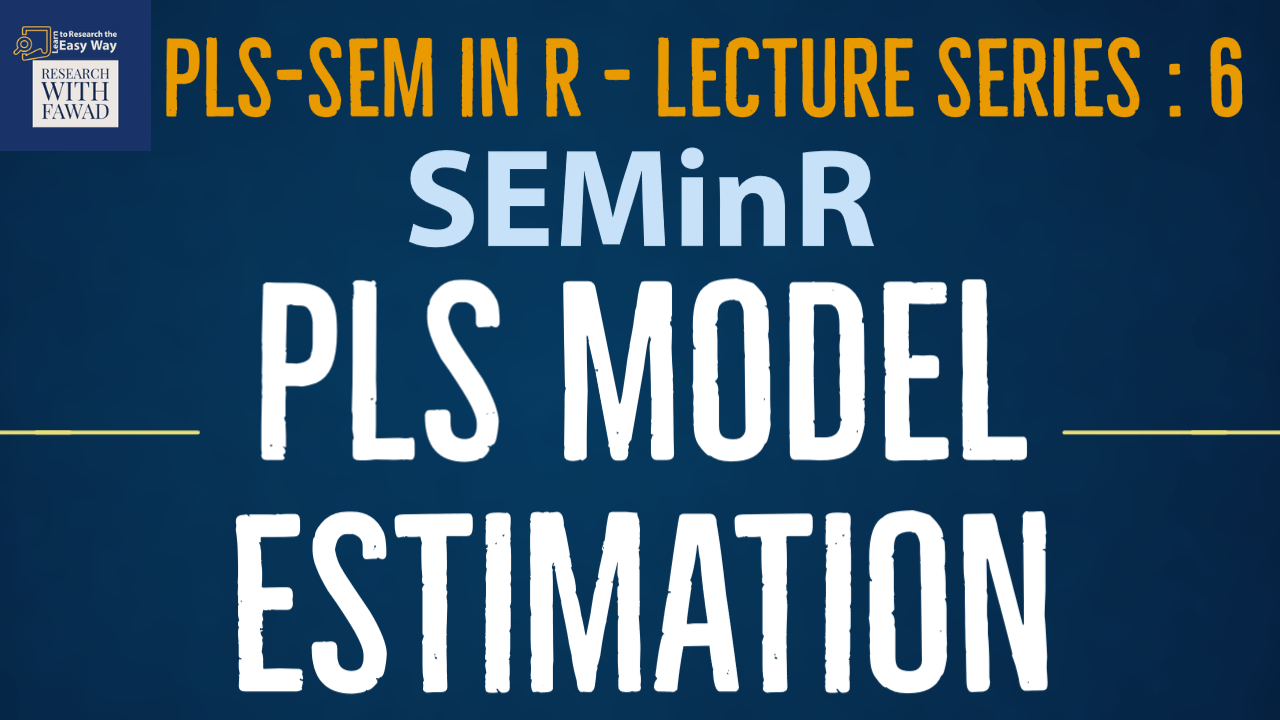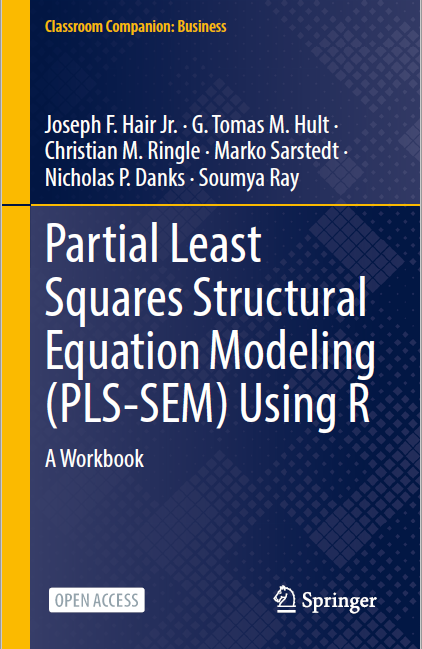# PLS Model Estimation in SEMinR### PLS Model Estimation in SEMinR

This tutorial on the SEMinR series will introduce how to estimate PLS moxel.

## SEMinR

There are four steps to specify and estimate a structural equation model using SEMinR:

## Step 4: Estimating the Model

Step 4 in creating a model

• After having specified the measurement and structural models, the next step is the model estimation using the PLS-SEM algorithm.
• For this task i-e estimation, the algorithm helps in determing the scores of the constructs that are later used as input for (single and multiple) regression models within the path model.
• After the algorithm has calculated the construct scores, the scores are used to estimate each regression model in the path model.
• As a result, we obtain the estimates for all relationships in the measurement models (i.e., the indicator weights/loadings) and the structural model (i.e., the path coefficients).
• To estimate a PLS path model, algorithmic options and argument settings must be selected. The algorithmic options and argument settings include selecting the structural model path weighting scheme. SEMinR allows the user to apply two structural model weighting schemes:
• The factor weighting scheme and
• The path weighting scheme.
• While the results differ little across the alternative weighting schemes, path weighting is the most popular and recommended approach.
• This weighting scheme provides the highest R-Sq value for endogenous latent variables and is generally applicable for all kinds of PLS path model specifications and estimations.
• SEMinR uses the estimate_pls() function to estimate the PLS-SEM model.
• This function applies the arguments shown in . Table. Please note that arguments with default values do not need to be specified but will revert to the default value when not specified.

• We now estimate the PLS-SEM model by using the estimate_pls() function with arguments

data = datas,

measurement_model = simple_mm,

structural_model = simple_sm,

inner_weights = path_weighting,

missing = mean_replacement, and

missing_value = “-99”

and assign the output to simple_model.

• It is like running PLS Algorithm in SmartPLS

``````# Estimate the model
simple_model <- estimate_pls(data = datas,
measurement_model = simple_mm,
structural_model = simple_sm,
inner_weights = path_weighting,
missing= mean_replacement,
missing_value = "-99")``````

Note that the arguments for inner_weights, missing, and missing_value can be omitted if the default arguments are used. This is equivalent to the previous code block:

``````# Estimate the model with Omissions
simple_model <- estimate_pls(data = datas,
measurement_model = simple_mm,
structural_model = simple_sm)``````

## Review of the Steps

Following is a brief review of the steps that have been discussed in SEMinR tutorials.

• Load the Library – library ()
• Review the Data – head()
• Specify the Measurement Model – constructs()
• Specify the Structural Model – relationships()
• Estimate the Model – estimate_pls()
• Summarize the Results – summary()
``````#Loading the Library
library(seminr)
#To Inspect Data
#Create measurement model
simple_mm <- constructs(
composite("Vision", multi_items("VIS", 1:4)),
composite("Development", multi_items("DEV", 1:7)),
composite("Rewards", multi_items("RW",1:4)),
composite("Collaborative Culture", multi_items("CC", 1:6)))
# Create structural model
simple_sm <- relationships(
paths(from = c("Vision", "Development", "Rewards"), to = "Collaborative Culture"))
# Estimate the model
simple_model <- estimate_pls(data = datas,
measurement_model = simple_mm, structural_model = simple_sm,
inner_weights = path_weighting,
missing = mean_replacement,
missing_value = "-99")
# Summarize the model results
summary_simple <- summary(simple_model)
#Inspect the Summary Report
summary_simple
# Inspect the model’s path coefficients and the R^2 values
summary_simple\$paths
# Inspect the construct reliability metrics
summary_simple\$reliability``````

## Reference

Hair Jr, J. F., Hult, G. T. M., Ringle, C. M., Sarstedt, M., Danks, N. P., & Ray, S. (2021). Partial Least Squares Structural Equation Modeling (PLS-SEM) Using R: A Workbook.The tutorials on SEMinR are based on the mentioned book. The book is open source and available for download under this link.